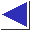# comp.lang.c FAQ list ·Question 3.11

Q: How can I avoid these undefined evaluation order difficulties if I don't feel like learning the complicated rules?

A: The easiest answer is that if you steer clear of expressions which don't have reasonably obvious interpretations, for the most part you'll steer clear of the undefined ones, too. (Of course, ``reasonably obvious'' means different things to different people. This answer works as long as you agree that a[i] = i++ and i = i++ are not ``reasonably obvious.'')

To be a bit more precise, here are some simpler rules which, though slightly more conservative than the ones in the Standard, will help to make sure that your code is ``reasonably obvious'' and equally understandable to both the compiler and your fellow programmers:

1. Make sure that each expression modifies at most one object. By ``object'' we mean either a simple variable, or a cell of an array, or the location pointed to by a pointer (e.g. *p). A ``modification'' is either simple assignment with the = operator, or a compound assignment with an operator like +=, -=, or *=, or an increment or decrement with ++ or -- (in either pre or post forms).
2. If an object (as defined above) appears more than once in an expression, and is the object modified in the expression, make sure that all appearances of the object which fetch its value participate in the computation of the new value which is stored. This rule allows the expression
```	i = i + 1
```
because although the object i appears twice and is modified, the appearance (on the right-hand side) which fetches i's old value is used to compute i's new value.
3. If you want to break rule 1, make sure that the several objects being modified are distinctly different, and try to limit yourself to two or at most three modifications, and of a style matching those of the following examples. (Also, make sure that you continue to follow rule 2 for each object modified.) The expression
```	c = *p++
```
is allowed under this rule, because the two objects modified (c and p) are distinct. The expression
```	*p++ = c
```
is also allowed, because p and *p (i.e. p itself and what it points to) are both modified but are almost certainly distinct. Similarly, both
```	c = a[i++]
and
a[i++] = c
```
are allowed, because c, i, and a[i] are presumably all distinct. Finally, expressions like
```	*p++ = *q++
```
and
```	a[i++] = b[j++]
```
in which three things are modified (p, q, and *p in the first expression, and i, j, and a[i] in the second), are allowed if all three objects are distinct, i.e. only if two different pointers p and q or two different array indices i and j are used.
4. You may also break rule 1 or 2 as long as you interpose a defined sequence point operator between the two modifications, or between the modification and the access. The expression
```	(c = getchar()) != EOF && c != '\n'
```
(commonly seen in a while loop while reading a line) is legal because the second access of the variable c occurs after the sequence point implied by &&. (Without the sequence point, the expression would be illegal because the access of c while comparing it to '\n' on the right does not ``determine the value to be stored'' on the left.)